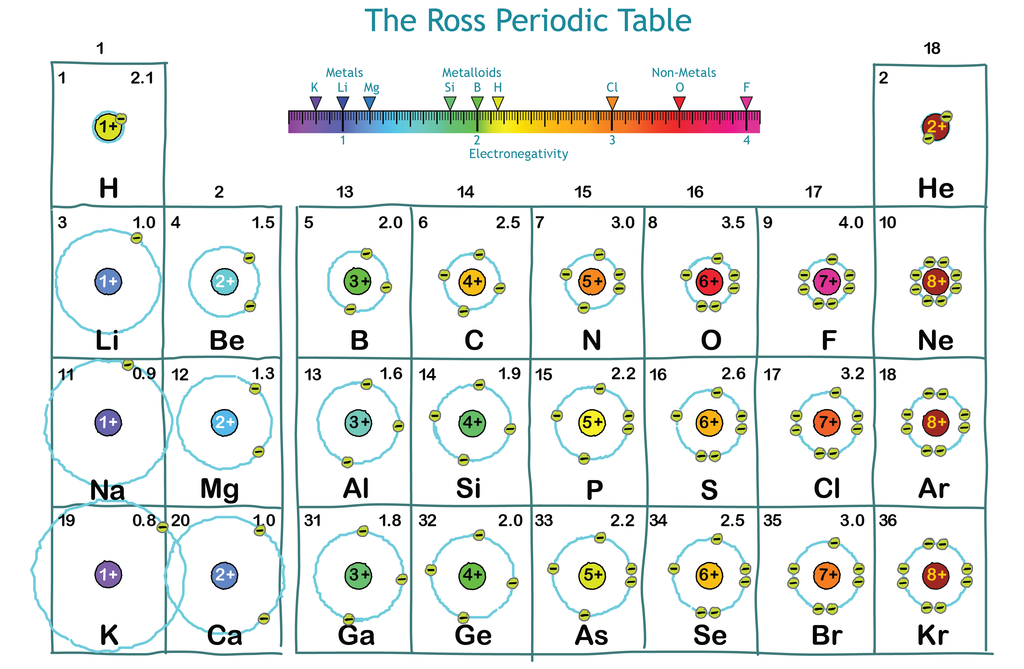# LP - What Makes Noble Gases Noble?

### This table exemplifies the design features of all IntuitivScience books.## This periodic table is designed for work!

### 1.   Extremely Easy to Learn

Start with a blank periodic table, with the same number of rows and columns as above. These are the main block elements. Your students can reproduce the rest of the table in five simple steps.

1. On Row 2, make a horizontal series of eight small circles to represent the atomic cores
2. Number the circles consecutively from 1+ to 8+ to represent the core charge
3. Draw a large circle around the first core to represent the valence orbit
4. Draw consecutively smaller valence circles from 1+ to 8+ atomic cores
5. Draw the correct number of valence electrons around the valence orbit. (The number of valence  electrons equals the core charge.)

That's it! Rows three and four are exactly the same, except that each valence shell is slightly larger in the lower rows.

### 2.   Only Three Salient FeaturesThe core charge, the radius, and the valence occupation are the three features that students use to make judgments about the chemical behavior of the elements.

They are also the three most visible features of each diagram.

Students don't have to wade through the details of a B-R atom.

### 3.   Students Intuitively Understand It.

To understand its chemistry, a student needs to consider only three facts about each atom.

1. The larger the core charge, the more strongly are the valence electrons are attracted.
2. The smaller the radius, the stronger the attractive force.
3. A full valence shell cannot receive more electrons.

It really is that easy. Students can easily grasp these three salient features of the Ross model. They can then use their intuitive schematic reasoning patterns to predict and explain the chemical behavior of each element.

For example, a student can intuitively see that a chlorine or oxygen atom, with a large core charge, a small radius, and one or two vacancies can attract and hold electrons from other atoms.

### 4.   Now Use the Table!Download the full-color table .pdf file, and print copies for your students.

1. Walk your students through the table, pointing out core charge.
2. Remove the table from view.
3. Ask them to make their own copy of the table on a piece of paper.

Most students will be able to draw the atomic cores with their positive charges, and the relative radius of each atom. Those few students who don't get it the first time, will probably be successful on the second try.

Lesson 1 - Learn the three salient features of each atom, and follow how they change across the periodic table.

Lesson 2 - Learn how students can make accurate predictions, with nearly no instruction!

Lesson 3 - Take a closer look at the elements of the second row.

Lesson 4 - Origins of the Ross model of the atom

Lesson 5 - Covalent bonding

Lesson 6 - Ionic Bonding

Lesson 7 - A post-modern model of science.

Lesson 8 - Learning with IntuitivScience.